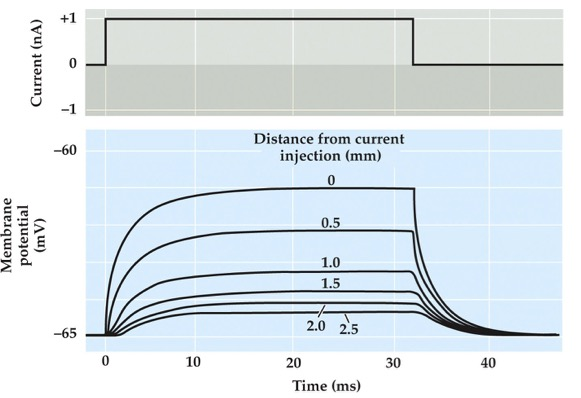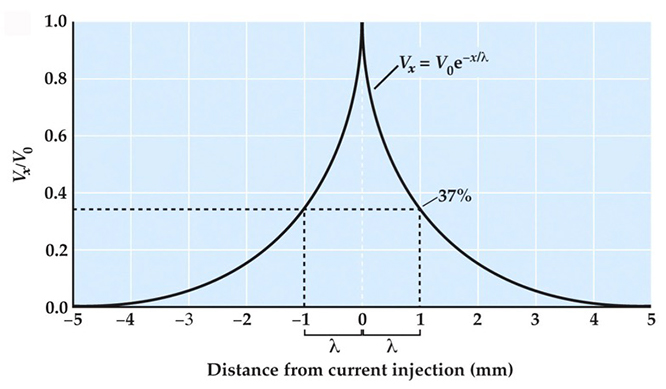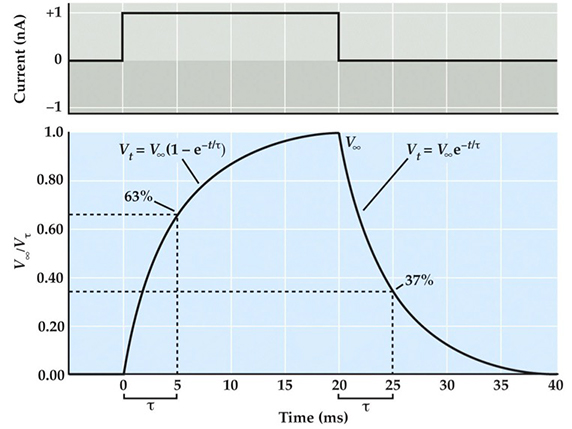# Neuroscience 6e Web Topic 2.1 - Passive Membrane Properties

The passive flow of electrical current plays a central role in action potential propagation, synaptic transmission, and all other forms of electrical signaling in nerve cells. Therefore, it is worthwhile understanding in quantitative terms how passive current flow varies with distance along a neuron. For the case of a cylindrical axon, such as the one depicted in Figure 2.3 in the textbook, subthreshold current injected into one part of the axon spreads passively along the axon until the current is dissipated by leakage out across the axon membrane. As a result of this leakage, potentials measured along the length of the axon get smaller with distance from the site where current is injected (Figure 1). The decrement in the current flow with distance can be determined by plotting the relative reduction in the amplitude of potentials over distance (Figure 2). This relationship is described by a simple exponential function:

$V_2=V_0 \,{e^{-x/\lambda}}$

where Vx is the voltage response at any distance x along the axon, V0 is the voltage change at the point where current is injected into the axon, e is the base of natural logarithms (approximately 2.7), and λ is the length constant of the axon. As is evident in this relationship, the length constant is the distance where the initial voltage response (V0) decays to 1/e (or 37%) of its value. The length constant is thus a way to characterize how far passive current flow spreads before it leaks out of the axon, with leakier axons having shorter length constants.Figure 1  Superimposed responses to a current pulse, measured at indicated distances along an axon.Figure 2  Spatial decay of membrane potential along a cylindrical axon. A current pulse injected at one point in the axon (0 mm) produces voltage responses (Vx) that decay exponentially with distance. The distance where the voltage response is 1/e of its initial value (V0) is the length constant, λ.

The length constant depends on the physical properties of the axon, in particular the relative resistances of the plasma membrane (rm), the intracellular axoplasm (ri), and the extracellular medium (r0). The relationship between these parameters is

$\sqrt{\frac{r_m}{r_o+r_i}}$

Hence, to improve the passive flow of current along an axon, the resistance of the plasma membrane should be as high as possible, and the resistances of the axoplasm and extracellular medium should be low.

Another important consequence of the passive properties of neurons is that currents flowing across a membrane do not immediately change the membrane potential. For example, when a rectangular current pulse is injected into the axon shown in the experiment illustrated in Figure 2.3A in the textbook, the membrane potential depolarizes slowly over a few milliseconds and then repolarizes over a similar time course when the current pulse ends (see Figure 1). These delays in changing the membrane potential are due to the fact that the plasma membrane behaves as a capacitor, storing the initial charge that flows at the beginning and end of the current pulse. For the case of a cell whose membrane potential is spatially uniform, the change in the membrane potential at any time, Vt, after beginning the current pulse (Figure 3) can also be described by an exponential relationship:

$V_t=V_\infty\left({1- e^{-t/\tau}}\right)$

where V∞ is the steady-state value of the membrane potential change, t is the time after the current pulse begins, and τ is the membrane time constant. The time constant is thus defined as the time when the voltage response (Vt) rises to 1 – (1/e) (or 63%) of V∞ . After the current pulse ends, the membrane potential change also declines exponentially according to the relationship

$V_t=V_\infty e^{-t/\tau}$

During this decay, the membrane potential returns to 1/e of V∞ at a time equal to τ. For cells with more complex geometries than that of the axon in Figure 2.3A in the textbook, the time courses of the changes in membrane potential are not simple exponentials, but nonetheless depend on the membrane time constant. Thus, the time constant characterizes how rapidly current flow changes the membrane potential.Figure 3  Time course of potential changes produced in a spatially uniform cell by a current pulse. The rise and fall of the membrane potential (V∞τ) can be described as exponential functions, with the time constant τ defining the time required for the response to rise to 1 – (1/e) of the steady-state value (V∞ ) or to decline to 1/e of V∞ .

The membrane time constant also depends on the physical properties of the nerve cell, specifically on the resistance (rm) and capacitance (cm) of the plasma membrane such that:

$\tau=r_mc_m$

The values of rm and cm depend, in part, on the size of the neuron, with larger cells having lower resistances and larger capacitances. In general, small nerve cells tend to have long time constants, and large cells tend to have brief time constants.

#### References

Hodgkin, A. L. and W. A. H. Rushton (1938) The electrical constants of a crustacean nerve fibre. Proc. R. Soc. Lond. 133: 444–479.

Johnston, D. and S. M.-S. WU (1995) Foundations of Cellular Neurophysiology. Cambridge, MA: MIT Press.

Rall, W. (1977) Core conductor theory and cable properties of neurons. In Handbook of Physiology, Section 1: The Nervous System, Vol. 1: Cellular Biology of Neurons. E. R. Kandel (ed.). Bethesda, MD: American Physiological Society, pp. 39–98.